# Logarithmic spiral facts for kids

Kids Encyclopedia Facts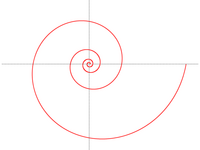Logarithmic spiral (pitch 10°)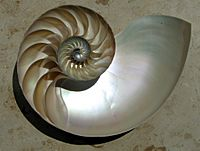Cutaway of a nautilus shell showing the chambers arranged in an approximately logarithmic spiral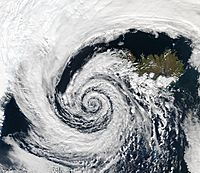A low pressure area over Iceland shows an approximately logarithmic spiral pattern.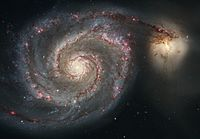The arms of spiral galaxies often have the shape of a logarithmic spiral, here the Whirlpool Galaxy.

A logarithmic spiral, equiangular spiral or growth spiral is a special kind of spiral curve which often appears in nature. The logarithmic spiral was first described by Descartes and later extensively investigated by Jakob Bernoulli, who called it Spira mirabilis, "the marvelous spiral".

## Definition

In polar coordinates (r, θ) the curve can be written as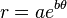$r = ae^{b\theta}\,$

or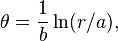$\theta = \frac{1}{b} \ln(r/a),$

hence the name "logarithmic". In parametric form, the curve is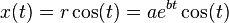$x(t) = r \cos(t) = ae^{bt} \cos(t)\,$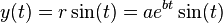$y(t) = r \sin(t) = ae^{bt} \sin(t)\,$

with real numbers a and b.

The spiral has the property that the angle ɸ between the tangent and radial line at the point (r,θ) is constant. This property can be expressed in differential geometric terms as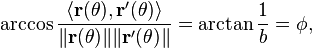$\arccos \frac{\langle \mathbf{r}(\theta), \mathbf{r}'(\theta) \rangle}{\|\mathbf{r}(\theta)\|\|\mathbf{r}'(\theta)\|} = \arctan \frac{1}{b} = \phi,$

The derivative r'(θ) is proportional to the parameter b. In other words, it controls how "tightly" and in which direction the spiral spirals. In the extreme case that b = 0 (ɸ = π/2) the spiral becomes a circle of radius a. Conversely, in the limit that b approaches infinity (ɸ → 0) the spiral tends toward a straight line. The complement of ɸ is called the pitch.

## Spira mirabilis and Jakob Bernoulli

Spira mirabilis, Latin for "miraculous spiral", is another name for the logarithmic spiral. While this curve had already been named by other mathematicians, the name "miraculous" or "marvelous" spiral was given to this curve by Jakob Bernoulli, because he was fascinated by one of its unique mathematical properties: the size of the spiral increases, but the shape stays the same with each added curve. Maybe because of this property, the spira mirabilis has evolved in nature, seen in some living beings, such as nautilus shells and sunflower heads. Jakob Bernoulli wanted the shape on his headstone, but, by error, an Archimedean spiral was placed there instead.

## Logarithmic spirals in nature

In several natural phenomena one may find curves that are close to being logarithmic spirals. Here follows some examples and reasons:

• The approach of a hawk to its prey. Their sharpest view is at an angle to their direction of flight; this angle is the same as the spiral's pitch.
• The approach of an insect to a light source. They are used to having the light source at a constant angle to their flight path. Usually the sun is the only light source and flying that way will result in a practically straight line.
• The arms of spiral galaxies. Our own galaxy, the Milky Way, is believed to have four major spiral arms, each of which is roughly a logarithmic spiral with pitch of about 12 degrees, an unusually small pitch angle for a galaxy such as the Milky Way. In general, arms in spiral galaxies have pitch angles ranging from about 10 to 40 degrees.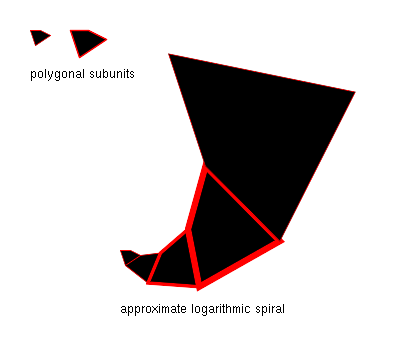• Many biological structures including spider webs and the shells of mollusks. In these cases, the reason is the following: Start with any irregularly shaped two-dimensional figure F0. Expand F0 by a certain factor to get F1, and place F1 next to F0, so that two sides touch. Now expand F1 by the same factor to get F2, and place it next to F1 as before. Repeating this will produce an approximate logarithmic spiral whose pitch is determined by the expansion factor and the angle with which the figures were placed next to each other. This is shown for polygonal figures in the accompanying graphic.

## Images for kidsLogarithmic spiral Facts for Kids. Kiddle Encyclopedia.